## Subtraction of Numbers Within 10000

Practice Unlimited Questions

#### 1. A fish tank has 3879 fishes. Another fish tank has 2836 fishes. What is the difference between the number of fishes in the two fish tanks?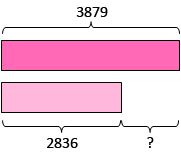Step 1: Subtract the ones.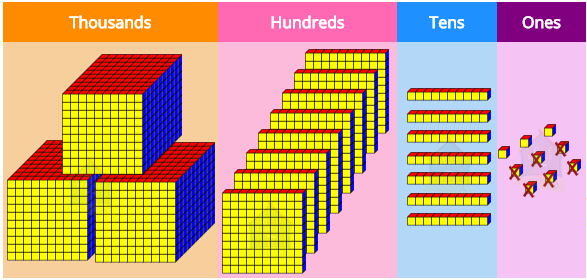9 ones − 6 ones
= 3 ones
Step 2: Subtract the tens.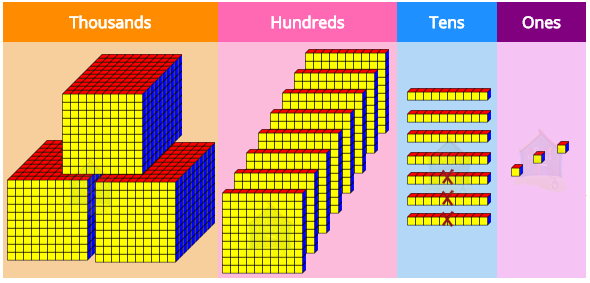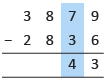7 tens − 3 tens
= 4 tens
Step 3: Subtract the hundreds.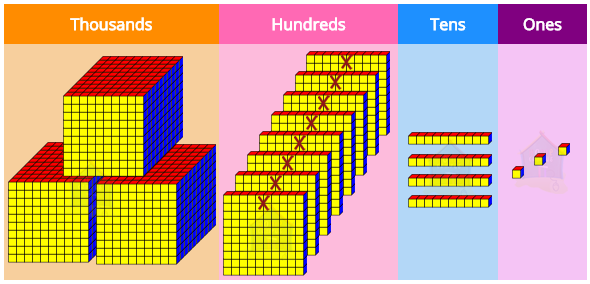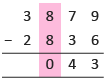8 hundreds − 8 hundreds
= 0 hundreds
Step 4: Subtract the thousands.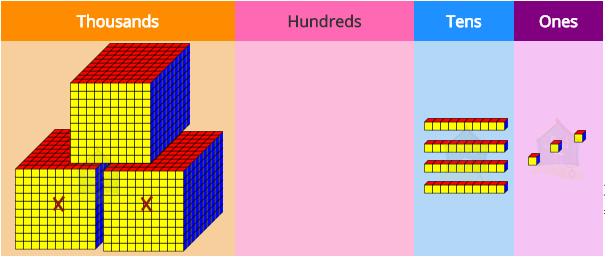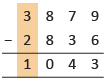3 thousands − 2 thousands
= 1 thousand
So, we get: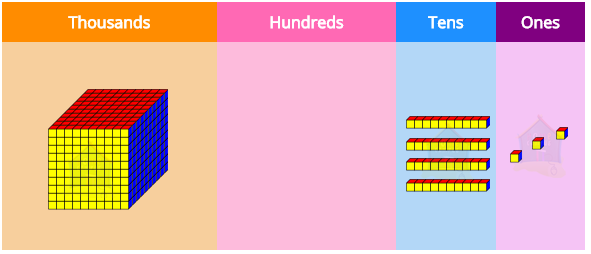The difference between the number of fishes in the two fish tanks is 1043.

#### 2. Jason has 4147 cards. Jordan has 2237 cards. How many more cards has Jason than Jordan?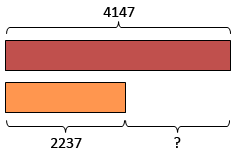Step 1: Subtract the ones.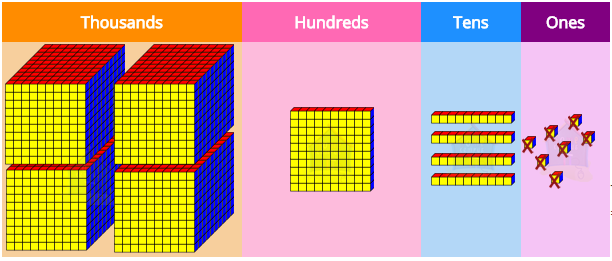7 ones − 7 ones
= 0 ones
Step 2: Subtract the tens.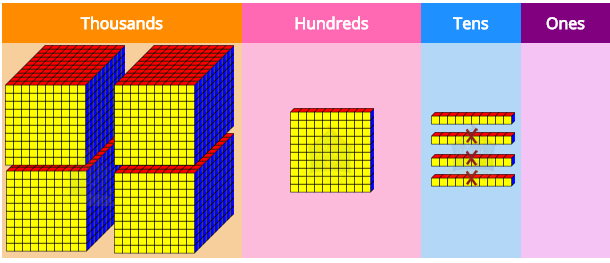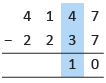4 tens − 3 tens
= 1 ten
Step 3a: Regroup.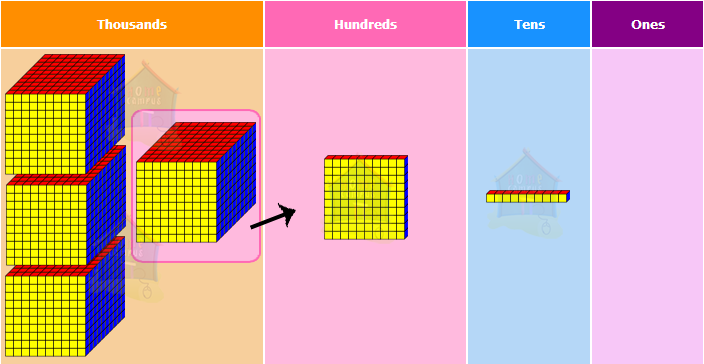2 hundreds cannot be subtracted from 1 hundred, so we regroup the thousands and the hundreds.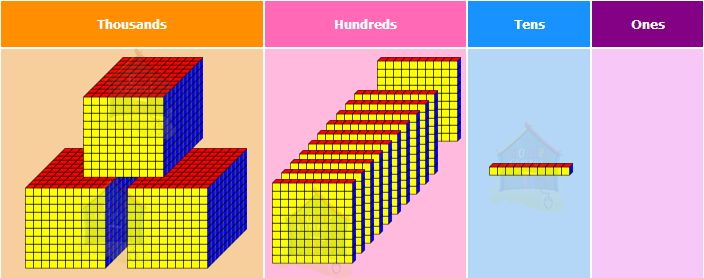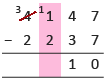4 thousands 1 hundred
= 3 thousands 11 hundreds
Step 3b: Subtract the hundreds.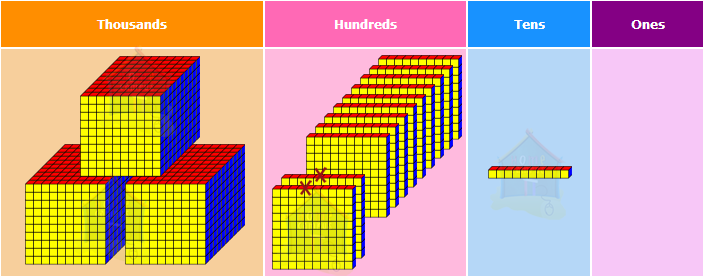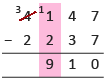11 hundreds − 2 hundreds
= 9 hundreds
Step 4: Subtract the thousands.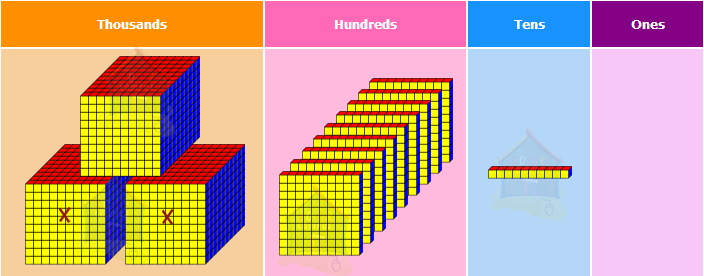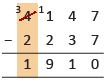3 thousands − 2 thousands
= 1 thousand
So, we get: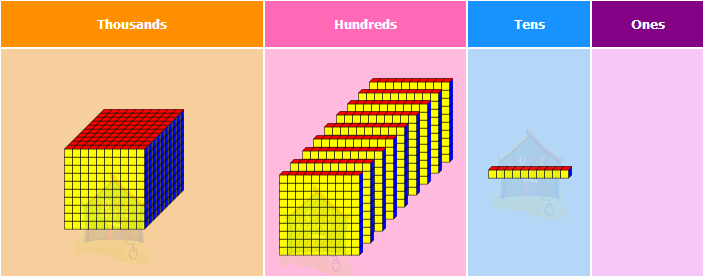Jason has 1910 more cards than Jordan.

#### 3. Susan and Anna had 3131 clips altogether. Susan had 1465 clips. Find the number of clips that Anna had.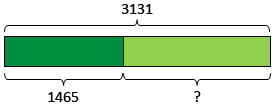Step 1a: Regroup the tens and the ones.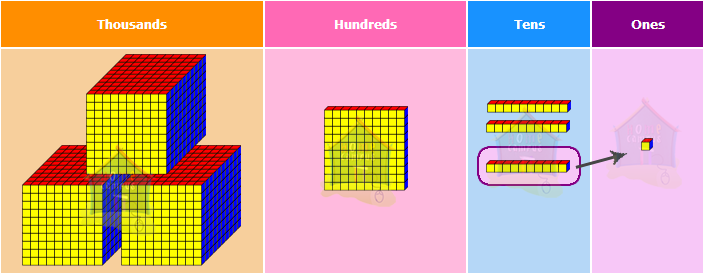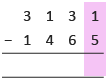5 ones cannot be subtracted from 1 one, so we regroup the tens and the ones.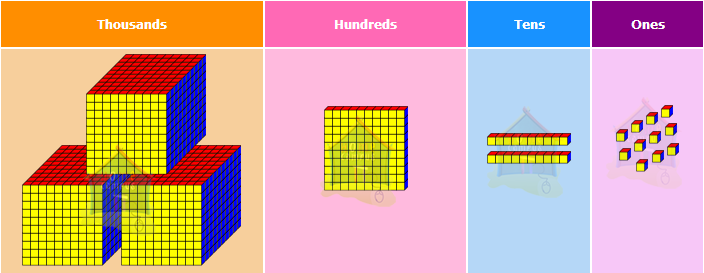3 tens 1 one
= 2 tens 11 ones
Step 1b: Now, subtract the ones.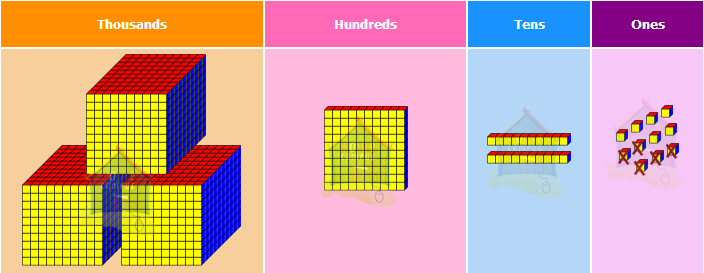11 ones − 5 ones
= 6 ones
Step 2a: Regroup the hundreds and the tens.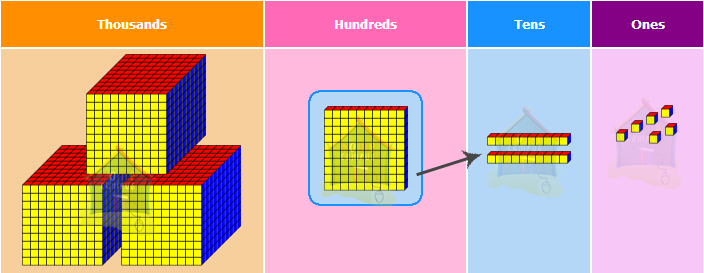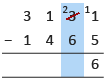6 tens cannot be subtracted from 2 tens, so we regroup the hundreds and the tens.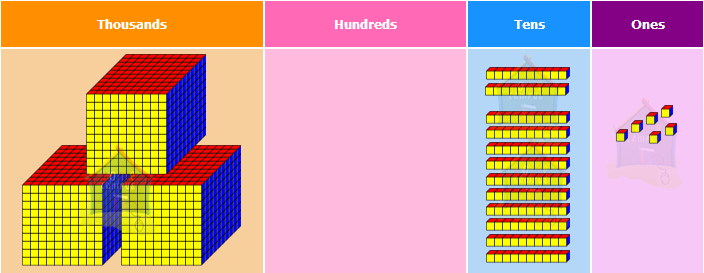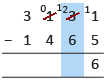1 hundred 2 tens
= 0 hundreds 12 tens
Step 2b: Now, subtract the tens.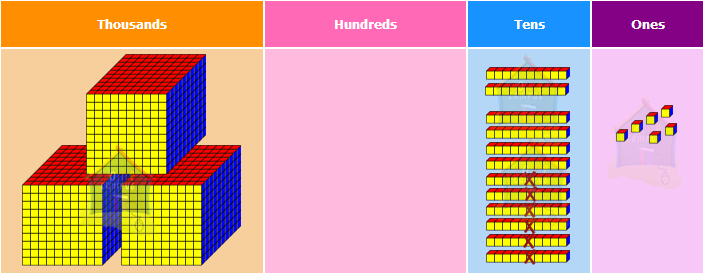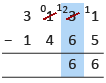12 tens − 6 tens
= 6 tens
Step 3a: Regroup the thousands and the hundreds.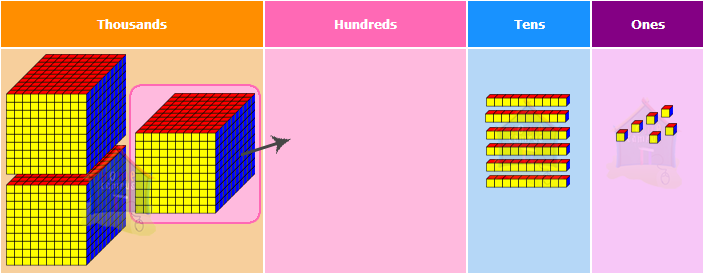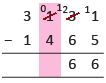4 hundreds cannot be subtracted from 0 hundreds, so we regroup the thousands and the hundreds.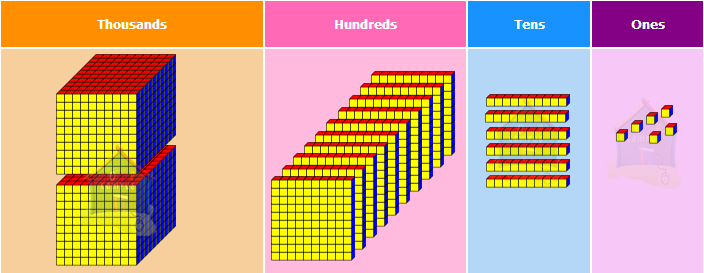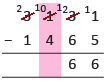3 thousands 0 hundreds
= 2 thousands 10 hundreds
Step 3b: Now, subtract the hundreds.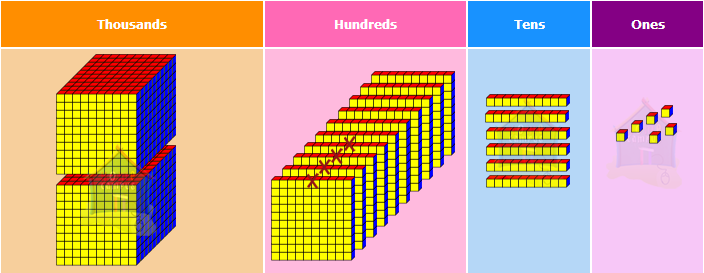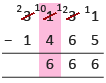10 hundreds − 4 hundreds
= 6 hundreds
Step 4: Subtract the thousands.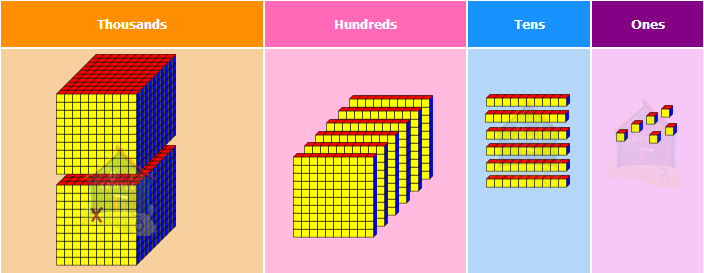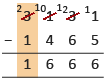2 thousands − 1 thousand
= 1 thousand
So, we get: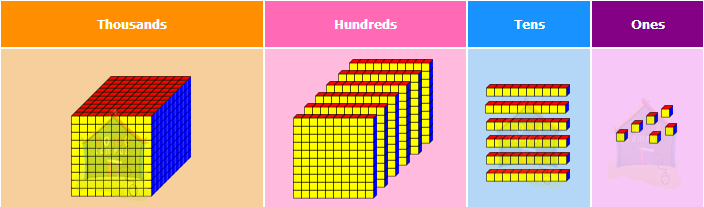Anna has 1666 clips.

#### 4. Mrs. Joseph earned \$4000. She spent \$2876 and saved the rest. How much money did she save?Step 1a: Regroup the thousands and hundreds.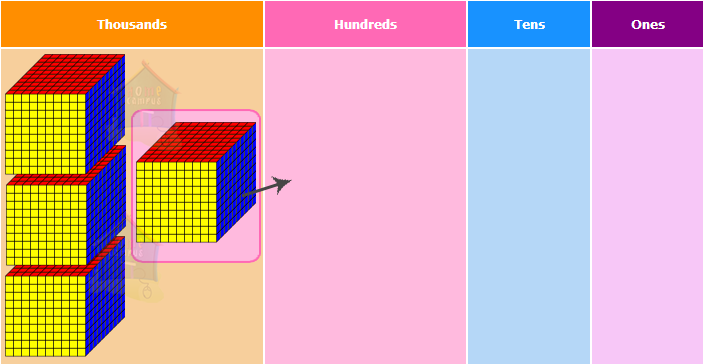Since the ones, tens and hundreds are all zeroes, we start by regrouping the thousands.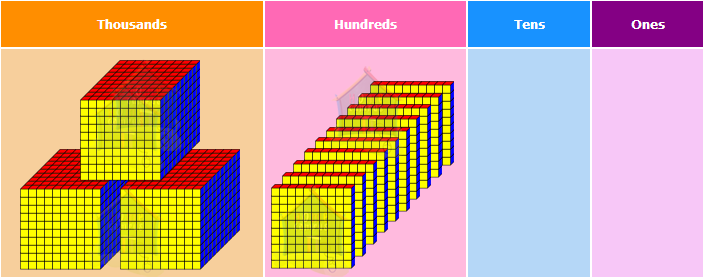3 tens 1 one
= 2 tens 11 ones
Step 1b: Regroup the hundreds and tens.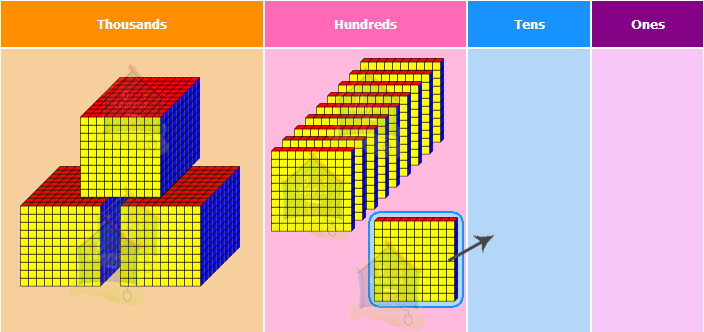Since the ones, tens and hundreds are all zeroes, we start by regrouping the thousands.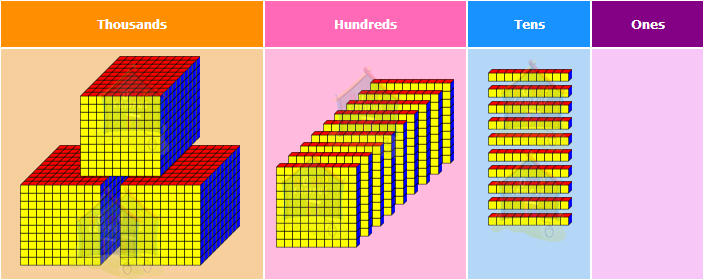10 hundreds
= 9 hundreds 10 tens
Step 1c: Regroup the tens and ones.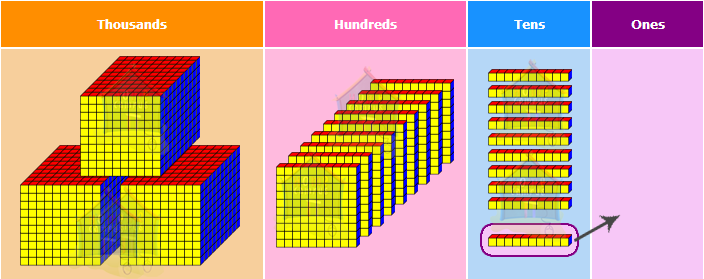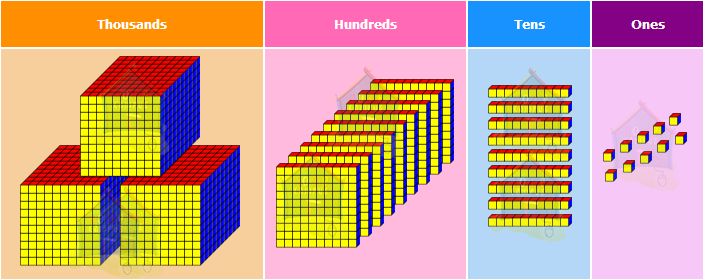10 tens
= 9 tens 10 ones
Step 1d: Now, subtract the ones.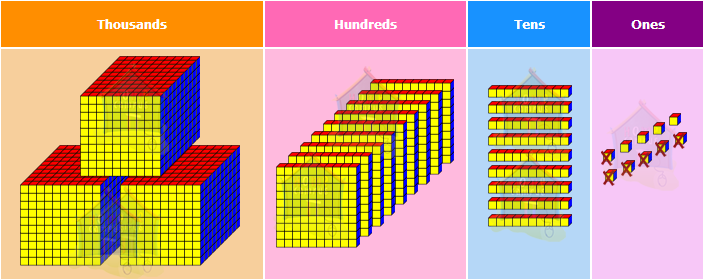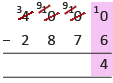10 ones − 6 ones
= 4 ones
Step 2: Next, subtract the tens.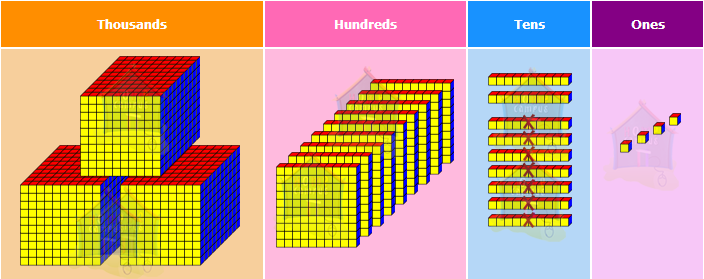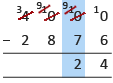9 tens − 7 tens
= 2 tens
Step 3: Then, subtract the hundreds.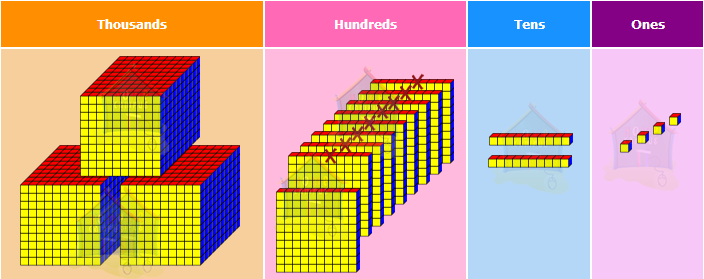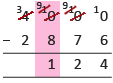9 hundreds − 8 hundreds
= 1 hundred
Step 4: Lastly, subtract the thousands.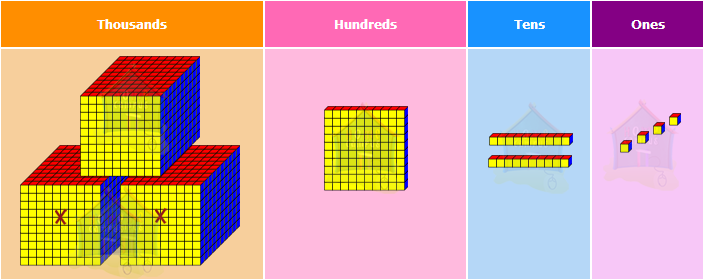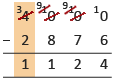3 thousands − 2 thousands
= 1 thousand
So, we get: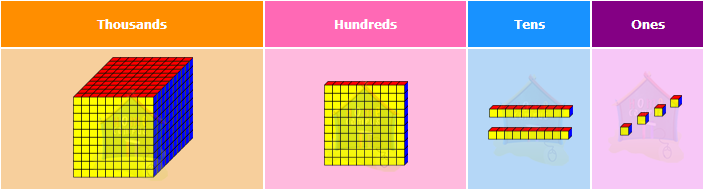She saved \$1124.

#### 5. Mr. Yap had 1354 mangoes. He sold 893 mangoes. How many mangoes had he left?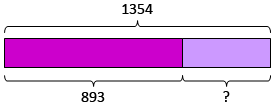1354  −  893  =  ?